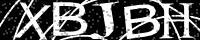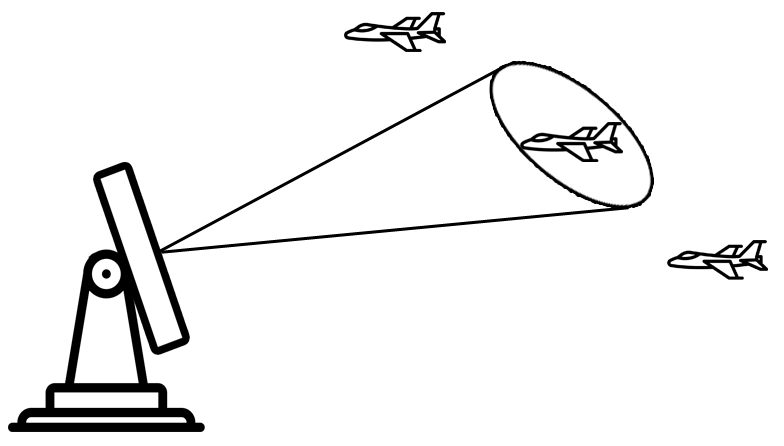# Overview

"If you can't explain it simply, you don't understand it well enough."

Albert Einstein

The Kalman Filter is an easy topic. However, many books and tutorials are not easy to understand. Most require extensive mathematical background and lack practical numerical examples. Almost every Kalman Filter book or tutorial makes it more complicated than necessary when the core idea is straightforward.

I decided to write a tutorial based on numerical examples with easy and intuitive explanations.

Some examples are from the radar world, where Kalman Filtering is used extensively (mainly for target tracking). However, the principles presented here can be applied to any field where estimation and prediction are required.

The tutorial includes three parts:

• Part 1 introduces the Kalman Filter topic. The introduction is based on eight numerical examples and doesn't require a priori mathematical knowledge. The tutorial provides all the necessary mathematical background, including terms such as mean, variance, and standard deviation. That is it. You can call it "The Kalman Filter for Dummies" if you like. After reading the first part, you will be able to understand the concept of the Kalman Filter and develop "Kalman Filter intuition." You will also be able to design a one-dimensional Kalman Filter.
• Part 2 describes a multidimensional (or multivariate) Kalman Filter - Kalman Filter in matrix notation. This part is a bit more advanced. Basic knowledge of Linear Algebra is required (only matrix operations). The necessary mathematical background is also provided in the tutorial. The mathematical derivation of the Kalman Filter and dynamic systems modeling are also included. After reading the second part, you will be able to understand the math behind the Kalman Filter. You will also be able to design a multidimensional Kalman Filter.
• Part 3 describes the non-linear Kalman Filter. This part is essential for mastering the Kalman Filter since most real-life systems are non-linear. Currently, this part is in the writing process. Part 3 will include Extended Kalman Filter, Unscented Kalman Filter, and Particle Filter.
I'll will never spam or share your email with anyone else."The road to learning by precept is long, by example short and effective."

Lucius Seneca

My name is Alex Becker. I am from Israel. I am an engineer with more than 20 years of experience in the Wireless Technologies field. As a part of my work, I had to deal with Kalman Filters, mainly for tracking applications.

Constructive criticism is always welcome. I would greatly appreciate your comments and suggestions. Please drop me an email.

The numerical examples in this tutorial do not exemplify any modes, methodologies, techniques, or parameters employed by any operational system known to the author.Most modern systems have numerous sensors that estimate hidden (unknown) states based on a series of measurements. For example, a GPS receiver provides location and velocity estimation, where location and velocity are the hidden states and differential time of the satellites' signals' arrival are the measurements.

One of the biggest challenges of tracking and control systems is providing an accurate and precise estimation of the hidden states in the presence of uncertainty. In GPS receivers, the measurement uncertainty depends on many external factors, such as thermal noise, atmospheric effects, slight changes in satellite positions, receiver clock precision, and many more.

The Kalman Filter is one of the most important and common estimation algorithms. The Kalman Filter produces estimates of hidden variables based on inaccurate and uncertain measurements. Also, the Kalman Filter predicts the future system state based on past estimations.

The filter is named after Rudolf E. Kálmán (May 19, 1930 – July 2, 2016). In 1960, Kálmán published his famous paper describing a recursive solution to the discrete-data linear filtering problem.

Today the Kalman filter is used in target tracking (Radar), location and navigation systems, control systems, computer graphics, and much more.## The prediction requirement

Before diving into the Kalman Filter explanation, let us first understand the need for a prediction algorithm.

As an example, let us consider a radar tracking algorithm.Assume a track cycle of 5 seconds. The tracking radar sends a pencil beam in the direction of the target. In other words, every 5 seconds, the radar revisits the target by sending a dedicated track beam in the direction of the target.

After sending the beam, the radar estimates the current target position and velocity. The radar also estimates (or predicts) the target position at the next track beam.

The future target position can be easily calculated using Newton's motion equations:

$x= x_{0} + v_{0} \Delta t+ \frac{1}{2}a \Delta t^{2}$
Where:
 $$x$$ is the target position $$x_{0}$$ is the initial target position $$v_{0}$$ is the initial target velocity $$a$$ is the target acceleration $$\Delta t$$ is the time interval (5 seconds in our example)

In three dimensions, Newton's motion equations can be written as a system of equations:

$\left\{\begin{matrix} x= x_{0} + v_{x0} \Delta t+ \frac{1}{2}a_{x} \Delta t^{2}\\ y= y_{0} + v_{y0} \Delta t+ \frac{1}{2}a_{y} \Delta t^{2}\\ z= z_{0} + v_{z0} \Delta t+ \frac{1}{2}a_{z} \Delta t^{2} \end{matrix}\right.$

The target parameters $$\left[ x, y, z, v_{x},v_{y},v_{z},a_{x},a_{y},a_{z} \right]$$ are called a System State. The current state is the input to the prediction algorithm, and the following state (the target parameters at the next time interval) is the algorithm output.

The above set of equations is called a Dynamic Model (or a State Space Model). The Dynamic Model describes the relationship between input and output.

Let us return to our example. As we can see, if the current state and the dynamic model are known, the next target state can be easily predicted.

Well, it is not. First of all, the radar measurement is not absolute. It includes a random error (or uncertainty). The error magnitude depends on many parameters, such as radar calibration, the beam width, and the signal-to-noise ratio of the returned echo. The error included in the measurement is called Measurement Noise.

Furthermore, the target motion is not strictly aligned with motion equations due to external factors such as wind, air turbulence, and pilot maneuvers. The dynamic model error (or uncertainty) is called Process Noise.

Due to Measurement Noise and Process Noise, the estimated target position can be far away from the actual target position. In this case, the radar might send the track beam in the wrong direction and miss the target.

We need a prediction algorithm that considers process and measurement uncertainty to improve the radar tracking performance.

The most widely used prediction algorithm is the Kalman Filter.